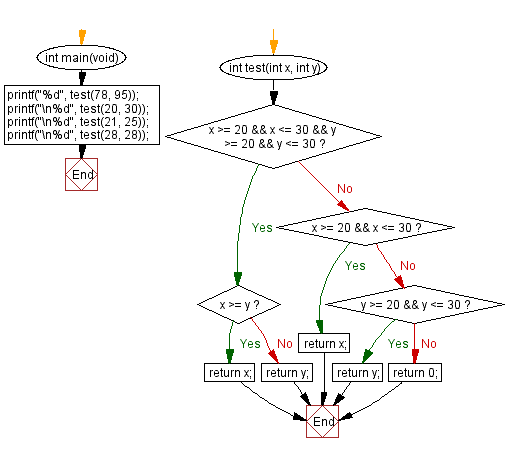﻿ C : In a range, find greater value, or 0 if neither exists# C Exercises: Find the larger value from two positive integer values that is in the range 20..30 inclusive, or return 0 if neither is in that range

## C-programming basic algorithm: Exercise-12 with Solution

Write a C program to find the larger value from two positive integer values that is in the range 20..30 inclusive, or return 0 if neither is in that range.

C Code:

``````#include <stdio.h>
#include <stdlib.h>
int main(void){
printf("%d",test(78, 95));
printf("\n%d",test(20, 30));
printf("\n%d",test(21, 25));
printf("\n%d",test(28, 28));
}
int test(int x, int y)
{
if (x >= 20 && x <= 30 && y >= 20 && y <= 30)
{
if (x >= y)
{
return x;
}
else
{
return y;
}
}
else if (x >= 20 && y <= 30)
{
return x;
}
else if (y >= 20 && y <= 30)
{
return y;
}
else
{
return 0;
}
}
``````

Sample Output:

```0
30
25
28
```

Pictorial Presentation:Flowchart:C Programming Code Editor:

What is the difficulty level of this exercise?

Test your Programming skills with w3resource's quiz.

﻿

## C Programming: Tips of the Day

Why doesn't a+++++b work?

printf("%d",a+++++b); is interpreted as (a++)++ + b according to the Maximal Munch Rule!.

++ (postfix) doesn't evaluate to an lvalue but it requires its operand to be an lvalue.

! 6.4/4 says the next preprocessing token is the longest sequence of characters that could constitute a preprocessing token"

Ref : https://bit.ly/3fdldUT